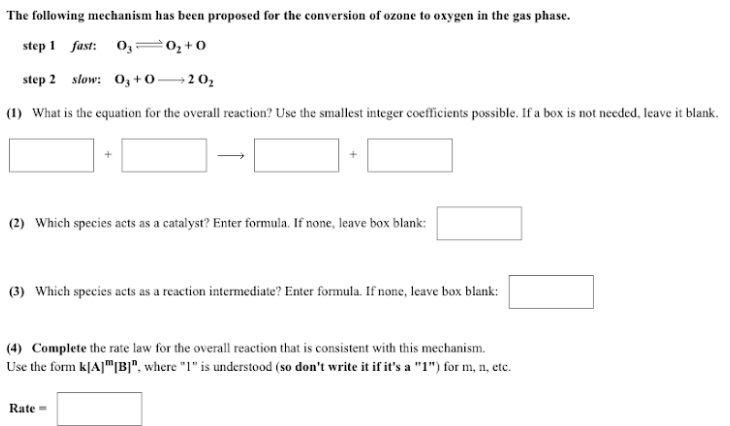# The following mechanism has been proposed for the conversion of ozone to oxygen in the gas phase. step 1 fast: O3 <-> O2 + O step 2 slow: O3 + O -> 2O2 1. What is the equation for the overall reaction? Use the smallest integer coefficients possible. 2. Which species acts as a catalyst? Enter formula. 3. Which species acts as a reaction intermediate? Enter formula. 4. Complete the rate law for the overall reaction that is consistent with this mechanism. Use the form k[A]^m[B]^n, where "1" is understood for m, n, etc. Rate =